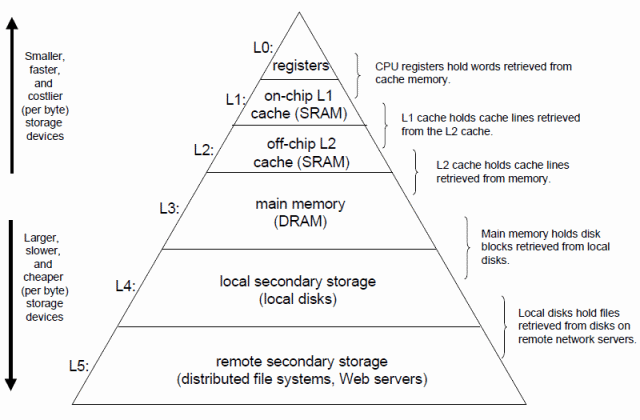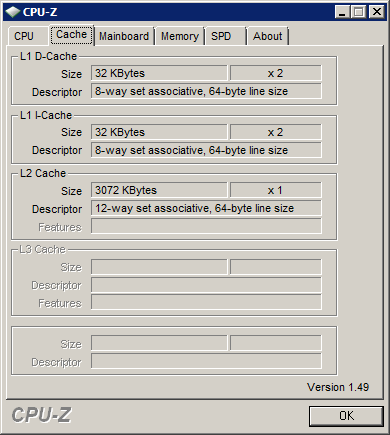## 计算机体系结构与程序性能

2009-01-22 08:28 by Jeffrey Zhao, ... 阅读, ... 评论, 收藏, 编辑

• 好多讲ASP.NET的文章啊，控件真好用，做网站很方便。
• ORM好像对开发很有帮助，我们也来LINQ to SQL，NHibernate一下……网站不就是CRUD吗？
• JavaScript框架XXX的效果好炫，网站越来越漂亮了。
• 好像进入并行处理时代了？呀，有了微软的并行库，加上AsParallel方法就能变快了。
• ……

（以上内容略有艺术夸张，如有雷同，绝非巧合）

# Locality

static void Main(string[] args)
{
int n = 1 << 10;
int[,] array = new int[n, n];

for (int x = 0; x < n; x++)
{
for (int y = 0; y < n; y++)
{
array[x, y] = x;
}
}

...
}


static int SumA(int[,] array, int n)
{
int sum = 0;
for (int y = 0; y < n; y++)
{
for (int x = 0; x < n; x++)
{
sum += array[x, y];
}
}

return sum;
}


static int SumB(int[,] array, int n)
{
int sum = 0;
for (int x = 0; x < n; x++)
{
for (int y = 0; y < n; y++)
{
sum += array[x, y];
}
}

return sum;
}


static void TestLocality(int[,] array, int n)
{
Stopwatch watch1 = new Stopwatch();
watch1.Start();
for (int i = 0; i < 100; i++) SumA(array, n);
watch1.Stop();
Console.WriteLine("SumA: " + watch1.Elapsed);

Stopwatch watch2 = new Stopwatch();
watch2.Start();
for (int i = 0; i < 100; i++) SumB(array, n);
watch2.Stop();
Console.WriteLine("SumB: " + watch2.Elapsed);
}

SumA: 00:00:04.8116776
SumB: 00:00:00.8342202

Locality（局部性，不知道该不该这么翻译），通俗地说，就是通过利用“缓存”来提高程序运行效率。缓存是计算机中无所不在的概念，这里先借用《Computer Systems: A Programmer's Perspective》（下文称为CSAPP）中的一幅图来简单说明一下：L1 Cache和L2 Cache的描述中都有“64-byte line size”字样，表示Cache的Line长为64字节。Line为Cache每次向下级存储设备读取数据的大小。例如，程序在运行时寄存器会向L1请求内存中某个地址的数据（可能是4字节），如果L1中没有这个地址的值，则会向L2中读取包含该地址的一整个Line的数据——也就是64字节，但是并不保证请求的4字节在这64字节的头部或尾部，CPU自有其对齐机制；如果L2没有这个地址的数据，则会向操作系统进行请求，同样是一个Line，64字节。

 0, 0 0, 1 0, 2 … … 0, 1021 0, 1022 0, 1023 1, 0 2, 0 … … 1021, 0 1022, 0 1023, 0 1023, 1 1023, 2 … … 1023, 1021 1023, 1022 1023, 1023

(0, 0), (0, 1), (0, 2), …, (0, 1022), (0, 1023), (1, 0), (1, 1), …, (1023, 0), (1023, 1), …, (1023, 1022), (1023, 1023)

1. 读取(0, 0)位置数据 => Cache Miss => L1向L2获取(0, 0), (0, 1), …, (0, 14), (0, 15)共64B数据 => 返回(0, 0)位置数据
2. 读取(1, 0)位置数据 => Cache Miss => L1向L2获取(1, 0), (1, 1), …, (1, 14), (1, 15)共64B数据 => 返回(1, 0)位置数据
3. ……
4. 读取(1023, 0)位置数据 => Cache Miss => L1向L2获取(1023, 0), (1023, 1), …, (1023, 14), (1023, 15)共64B数据 => 返回(1023, 0)位置数据
5. 读取(0, 1)位置数据 => Cache Miss（因为L1大小有限，读取(0, 0)时放入L1的64B数据已经被其他数据替换） => L1向L2获取(0, 0), (0, 1), …, (0, 14), (0, 15)共64B数据 => 返回(0, 1)位置数据
6. 读取(1, 1)位置数据 => Cache Miss（理由同上） => L1向L2获取(1, 0), (1, 1), …, (1, 14), (1, 15)共64B数据 => 返回(1, 1)位置数据
7. ……

1. 读取(0, 0)位置数据 => Cache Miss => L1向L2获取(0, 0), (0, 1), …, (0, 14), (0, 15)共64B数据 => 返回(0, 0)位置数据
2. 读取(0, 1)位置数据 => Cache Hit => 直接返回(0, 1)位置数据
3. 读取(0, 2)位置数据 => Cache Hit => 直接返回(0, 2)位置数据
4. ……
5. 读取(1, 0)位置数据 => Cache Miss => L1向L2获取(1, 0), (1, 1), …, (1, 14), (1, 15)共64B数据 => 返回(1, 0)位置数据
6. 读取(1, 1)位置数据 => Cache Hit => 直接返回返回(1, 1)位置数据
7. ……

# False Sharing

static int ParallelSumA(int[,] array, int n)
{
int processorCount = Environment.ProcessorCount;
int[] result = new int[processorCount];

Parallel.For(0, processorCount, (part) =>
{
int minInclusive = part * n / processorCount;
int maxExclusive = minInclusive + n / processorCount;

for (int x = minInclusive; x < maxExclusive; x++)
{
for (int y = 0; y < n; y++)
{
result[part] += array[x, y];
}
}
});

int sum = 0;
for (int i = 0; i < result.Length; i++)
{
sum += result[i];
}

return sum;
}


00:00:01.8105218

False Sharing（错误共享）意为错误地共享了本不该共享的数据。由于每个核的L1缓存互相独立，因此CPU必须有一种机制，能够确保一个核在向它的L1 Cache中写入一个值之后，其他核内L1 Cache中包含这个数据的整个Line就会过期。这意味着其他核在读取地址相同，或者是接近的数据时会遇到L1 Cache Miss。CPU的这种同步机制就是MESI协议。那么我们来分析一下上面的代码到底如何造成了False Sharing。

1. CL0读取result的值 => Cache Miss => result数组被加载到CL0中 => 修改CL0中result
2. CL1读取result的值 => Cache Miss => result数组被加载到CL1中 => 修改CL1中result
3. CL0读取result的值 => 由于刚才CL1修改了result，导致整条Line失效，于是Cache Miss => result数组被加载到CL0中 => 修改CL0中result
4. CL1读取result的值 => 由于刚才CL0修改了result，导致整条Line失效，于是Cache Miss => result数组被加载到CL1中 => 修改CL0中result
5. ……

static int ParallelSumB(int[,] array, int n)
{
int processorCount = Environment.ProcessorCount;
int[] result = new int[processorCount];

Parallel.For(0, processorCount, (part) =>
{
int partSum = 0;
int minInclusive = part * n / processorCount;
int maxExclusive = minInclusive + n / processorCount;

for (int x = minInclusive; x < maxExclusive; x++)
{
for (int y = 0; y < n; y++)
{
partSum += array[x, y];
}
}

result[part] = partSum;
});

int sum = 0;
for (int i = 0; i < result.Length; i++)
{
sum += result[i];
}

return sum;


00:00:00.5366263